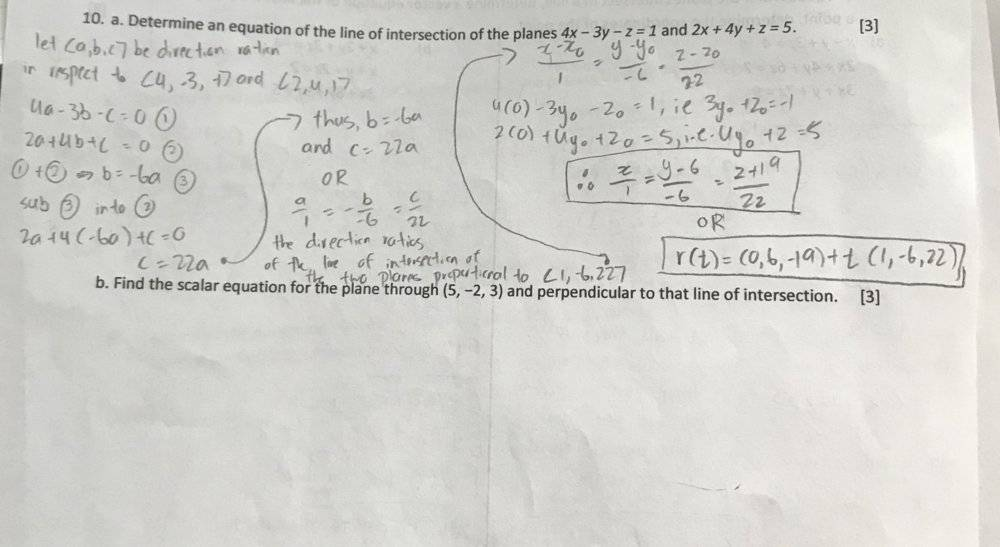# Finding the Scalar Equation for a Plane through a Point and Perpendicular to a Line

Homework Statement:
a. Determine an equation of the line of intersection of the planes 4x − 3y − z = 1 and 2x + 4y + z =5.
b. Find the scalar equation for the plane through (5, −2, 3) and perpendicular to that line of intersection.

I solved a, I'm not sure how to attempt b :/
Relevant Equations:
n/aLast edited by a moderator:

## Answers and Replies

Mark44
Mentor
ttpp1124 said:
b. Find the scalar equation for the plane through (5, −2, 3) and perpendicular to that line of intersection.
You have an equation of the line of intersection of the two planes. The plane you're looking for in part b is perpendicular to the line of intersection, thus a normal to that plane is parallel to the line you found.
It shouldn't be difficult to find an equation of a plane if you know 1) a point on the plane, and 2) a normal to that plane.
For part a, did you check your work? Any point on the line should be on both planes, and the direction vector of the line should be perpendicular to the normal of the two planes.

You have an equation of the line of intersection of the two planes. The plane you're looking for in part b is perpendicular to the line of intersection, thus a normal to that plane is parallel to the line you found.
It shouldn't be difficult to find an equation of a plane if you know 1) a point on the plane, and 2) a normal to that plane.
For part a, did you check your work? Any point on the line should be on both planes, and the direction vector of the line should be perpendicular to the normal of the two planes.
I did check my work for part a, I'm confident in my answer.
Since 𝐍=(1,−6,22)N=(1,−6,22) is a normal to the plane its equation is
𝐍⋅(𝑥,𝑦,𝑧)−(5,−2,3))=0.

Mark44
Mentor
I did check my work for part a, I'm confident in my answer.
Since 𝐍=(1,−6,22)N=(1,−6,22) is a normal to the plane its equation is
𝐍⋅(𝑥,𝑦,𝑧)−(5,−2,3))=0.
But don't leave it in that form, since that problem asks for the scalar equation of the plane. This will be either Ax + By + Cz = D or ##A(x - x_0) + B(y - y_0) + C(z - z_0) = 0##, where ##(x_0, y_0, z_0)## is the known point and <A, B, C> is a normal to the plane.

Mark44
Mentor
1(x-5)-6(y+2)+22(z-3)
That's not an equation.

That's not an equation.
can you tell me where I went wrong?

EDIT: 𝑥−6𝑦+22𝑧=83 is my answer.

Mark44
Mentor
can you tell me where I went wrong?

EDIT: 𝑥−6𝑦+22𝑧=83 is my answer.
That's better.